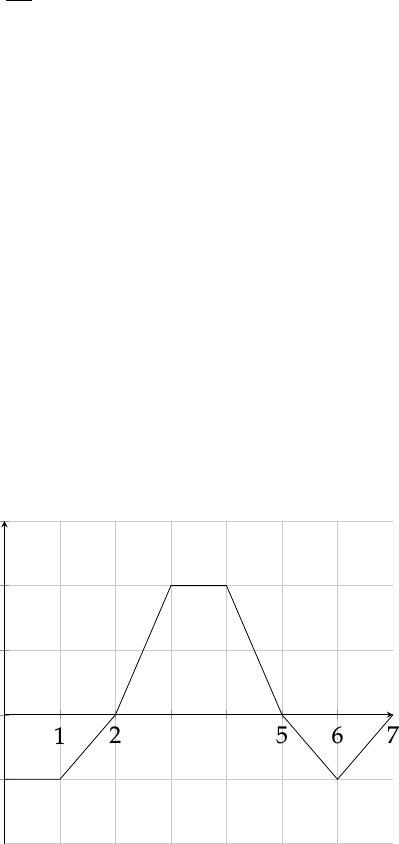Study Guides (390,000)
US (220,000)
UC-Irvine (3,000)
MATH (600)
MATH 2B (100)
All (60)
Midterm

# MATH 2B Midterm: MATH 2B Midterm Fall 2014Exam

Department
Mathematics
Course Code
MATH 2B
Professor
All
Study Guide
Midterm

This preview shows page 1. to view the full 5 pages of the document.Fall 2014 Math 2B - First Midterm
This exam consists of 5 questions. Problems #1 and 4 are worth 15 points each, problem
#2 is worth 10 points and problems #3 and 5 are worth 25 points each. Read directions for
each problem carefully. Please show all work needed to arrive at your solutions. Clearly
Name :
Problem 1 : Determine whether the statement is true or false. If it is true, explain
why. If it is false, give the right result or an example that disproves the statement.
(a) If fis continuous, d
dx Zb
af(x)dx=f(x).
(b) If Z1
0f(x)dx =0, then f(x) = 0 for 0 x1.
(c) Below is the graph of the function g. Let f(x) = Rx
0g(t)dt for all 0 x7.
12 3 4567
2
1
0
1
2
3
1

Unlock to view full version

Only page 1 are available for preview. Some parts have been intentionally blurred.(i) fhas a local maximum at x=5.
(ii) f(3) = 5
2.
(iii) f(2) = 0.
Problem 2 : (a) Use three rectangles and the midpoint rule to approximate the
area under the graph f(x) = 1
2x+1 and above the x-axis from x=1 to x=7.
(b) Find an expression for the same area as a limit of a Riemann sum. (You do
not need to evaluate it.)
2